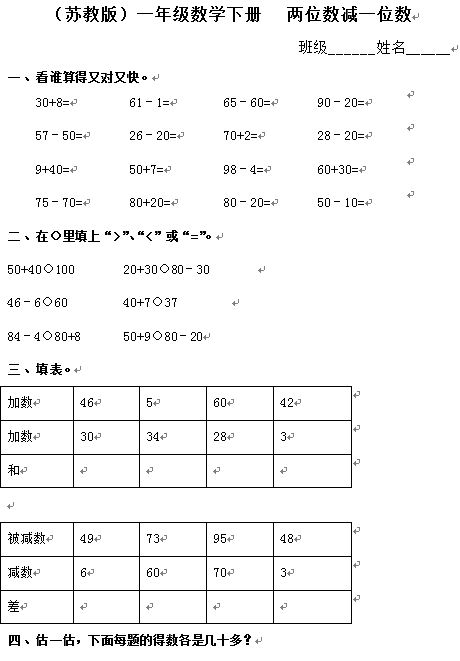（苏教版）一年级数学下册   两位数减一位数

 30+8= 61－1= 65－60= 90－20= 57－50= 26－20= 70+2= 28－20=

【DOC文档4页】（苏教版）一年级数学下册 两位数减一位数及答案 2 A4电子版资料_可直接打印_会员免费下载评论信息